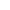# CASIO fx-83GT PLUS complex numbers output problem

casio casio fx-83GT PLUS CASIO fx Complex numbers AnswerBest Answer pan.gejt , 26 November 2016 - 07:57 PM

Recall X and Y to get the magnitude and the angle.

Math notice: Your result is incorrect. This operation (400)/(Pol(0.7-15,1.9+1.9)) is not defined because you cannot perform calculation "constant/(magnitude,angle)". How you can divide the magnitude and the angle simultaneously? You can perform Pol(X, Y) only. You enter 2 parameters and your result is also 2 parameters.

The magnitude is 14.8 and the angle is 165.1 degree.

Go to the full post2 replies to this topic

### #1duder5234

duder5234

Newbie

•• Members
•• 2 posts

• Calculators:
CASIO fx-83GT PLUS

Posted 26 November 2016 - 02:30 PM

Hello,

So I am having a problem with the output of my CASIO fx-83GT PLUS, I have tried a lot of things, but I can't get the correct output. This is what I am trying to input...

(400)/(Pol(0.7-15,1.9+1.9))

The answer should give the magnitude and the angle, but for some reason the calculated angle does not appear on the screen, only the magnitude. So the answer shown on my calculator is just: 27.03381595

Is there any way to solve this? Thank you.

### #2pan.gejt

pan.gejt

Casio Freak

•• Members
•• 205 posts
• Gender:Male
• Location:CZ

• Calculators:
.

Posted 26 November 2016 - 07:57 PM   Best Answer

Recall X and Y to get the magnitude and the angle.

Math notice: Your result is incorrect. This operation (400)/(Pol(0.7-15,1.9+1.9)) is not defined because you cannot perform calculation "constant/(magnitude,angle)". How you can divide the magnitude and the angle simultaneously? You can perform Pol(X, Y) only. You enter 2 parameters and your result is also 2 parameters.

The magnitude is 14.8 and the angle is 165.1 degree.

• duder5234 likes this

### #3duder5234

duder5234

Newbie

•• Members
•• 2 posts

• Calculators:
CASIO fx-83GT PLUS

Posted 26 November 2016 - 09:53 PM

Recall X and Y to get the magnitude and the angle.

Math notice: Your result is incorrect. This operation (400)/(Pol(0.7-15,1.9+1.9)) is not defined because you cannot perform calculation "constant/(magnitude,angle)". How you can divide the magnitude and the angle simultaneously? You can perform Pol(X, Y) only. You enter 2 parameters and your result is also 2 parameters.

The magnitude is 14.8 and the angle is 165.1 degree.

Hi Casio Freak,

That explains a lot! Thank you for the explanation, I am not too good with complex numbers, as you can see! This whole time I thought the calculator just wasn't able to compute the calculation, but it was just me being stupid. Cheers again, and have a good day.

### Also tagged with one or more of these keywords: casio, casio fx-83GT PLUS, CASIO fx, Complex numbers, Answer

#### 0 user(s) are reading this topic

0 members, 0 guests, 0 anonymous users